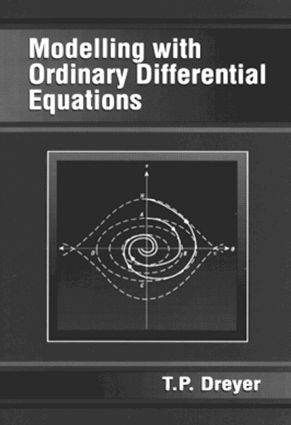# Modelling with Ordinary Differential Equations

## 1st Edition

CRC Press

304 pages

##### Purchasing Options:\$ = USD
Paperback: 9780849386367
pub: 1993-06-28
SAVE ~\$18.99
\$94.95
\$75.96
x
Hardback: 9781138417540
pub: 2017-07-27
SAVE ~\$41.00
\$205.00
\$164.00
x
eBook (VitalSource) : 9780203746790
pub: 2017-09-06
from \$47.48

FREE Standard Shipping!

### Description

Modelling with Ordinary Differential Equations integrates standard material from an elementary course on ordinary differential equations with the skills of mathematical modeling in a number of diverse real-world situations. Each situation highlights a different aspect of the theory or modeling. Carefully selected exercises and projects present excellent opportunities for tutorial sessions and self-study.

This text/reference addresses common types of first order ordinary differential equations and the basic theory of linear second order equations with constant coefficients. It also explores the elementary theory of systems of differential equations, Laplace transforms, and numerical solutions. Theorems on the existence and uniqueness of solutions are a central feature. Topics such as curve fitting, time-delay equations, and phase plane diagrams are introduced. The book includes algorithms for computer programs as an integral part of the answer-finding process. Professionals and students in the social and biological sciences, as well as those in physics and mathematics will find this text/reference indispensable for self-study.

Introduction: Mathematical Modelling. Boundary Value Problems. Direction Fields. Finding Zeros. Exercises.

First Order Differential Equations: Population Growth: The Malthus Model. Least Squares Method of Curve Fitting. Population Growth: The Logistic Model. Harvesting. Optimization of Profit. Epidemics. Potato Blight. Free Fall with Air Resistance. Power. Rockets. Exercises. Projects. Mathematical Background.

Numerical Methods: Existence Theorem. Euler Algorithm. Error Analysis. Runge-Kutta Algorithm. Fruitflies. Exercises. Projects. Mathematical Background.

Laplace Transforms: Linear Transforms. Properties of Laplace Transforms. Solving Differential Equations with Laplace Transforms. Table of Laplace Transforms. Exercises. Projects. Mathematical Background.

Simultaneous Linear First Order Differential Equations: Projectile Trajectories with Air Resistance. Romantic Relationships. Neutron Flow. Electrical Networks. Marriage. Residential Segregation. Exercises. Projects. Mathematical Background.

Second Order Linear Differential Equations: Mechanical Vibrations. Electrical Networks. The Ignition System of an Automobile. Simultaneous Equations. Exercises. Projects. Mathematical Background.

Non-Linear Second Order Differential Equations: The Pendulum without Damping. The Pendulum with Damping. Population of Interacting Species. Exercises. Mathematical Background. Table of Integrals. Answers. References. Index.

### Subject Categories

##### BISAC Subject Codes/Headings:
MAT000000
MATHEMATICS / General
MAT003000
MATHEMATICS / Applied
MAT007000
MATHEMATICS / Differential Equations